# Harmonic Forced Vibration of a Plane GridThis test lets you check analysis results for a plane grid, in the context of an harmonic dynamic response case. You will use a 1D mesh.

This test is used to validate the following attributes:

• 1D beam elements

• Harmonic dynamic response solve algorithms.Reference:

AFNOR technique, SFM Paris, Guide de validation des progiciels de calcul de structures, SSLL 04/89, pp.198-199, 1990.

##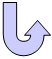Specifications

### Geometry Specifications

 Length: L1 = L2 = 5 m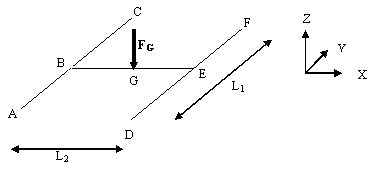Section IPE 200 : area = 2.872e-3 m2 Iy = 1.943e-5 m4 Iz = 1.424e-6 m4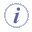The geometry is built with two Parts, inserted in a Product. The first Part includes the beam AC and the beam DF. The second Part includes the beam BE.

### Analysis Specifications

 Young Modulus (material) E = 200 GPa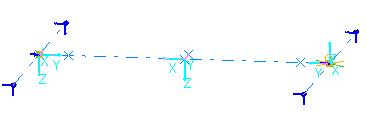Poisson's Ratio (material) ν = 0.3 Density:  ρ = 7800 kg/m3 Mesh Specifications: 500 mm elements for the three edges No automatic mesh capture for all For ball joints B and E, rigid connection property with transmitted degrees of freedom Tx = Ty = Tz = 0 Restraints (User-defined): At points A, C, D and F : Tx = Ty = Tz = 0 Edges AC and DF : Ry = 0 Edge BE : Rx = 0 Loads: F = F0 sin ωt Distributed force F0 = -100 000 N ω = 80 rad/s, so f = 12.7324 Hz

##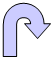Results

### Frequency Response

 Fundamental mode Frequency [Hz] Error [%] Analytical solution Computed results 1 16.456 16.410 0.28 2 38.165 37.941 0.59

### Harmonic Dynamic Response

Displacement on z axis at f = 12.7324 Hz

 Point Type of values Analytical solution Computed results Error [%] B and E WBmax [mm] -98.00 -100.35 2.4 G WG+WGmax [mm] -227.00 -226.50 0.2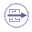To Perform the Test:

The Harmonic_forced_vibrations_plane_grid.CATAnalysis document presents a complete analysis of this case.

Proceed as follow:

1. Open the CATAnalysis document.

2. Compute the case and generate an image called Deformed mesh.